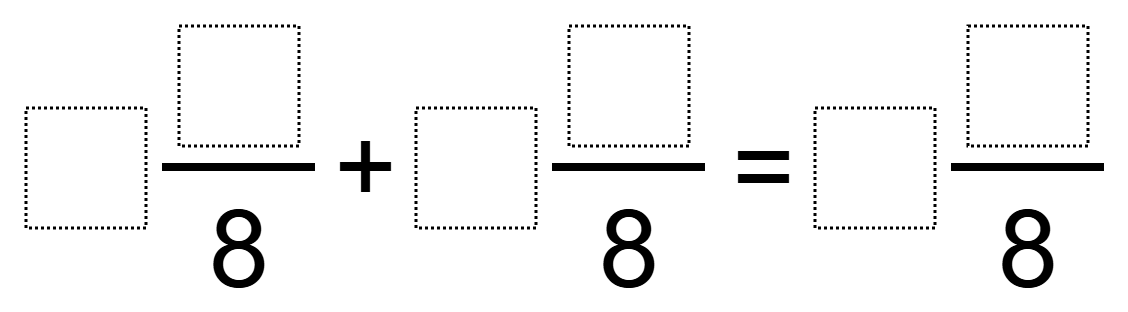Directions: Using the digits 1 to 9 at most one time each, place a digit in each box to make a true equation with the smallest possible sum.### Hint

Which digits should you use/avoid? Where should you put the 1? The 2? The…